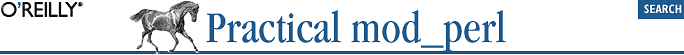home | O'Reilly's CD bookshelfs | FreeBSD | Linux | Cisco | Cisco Exam## 13.12. Comparing Runtime Performance of Perl and C

We know we can use the Benchmark module to compare Perl code. There are equivalent tools for C also, but how are we going to use two different tools and keep the comparison fair? Since Perl is a glue language in addition to its own merits, we can glue the C code into Perl and then use the Benchmark module to run the benchmark.

To simplify the task, we are going to demonstrate only the fact that C is more suitable than Perl for mathematical and memory-manipulation tasks. The purpose is to show how to use the best of both worlds.

We will use a very simple task that we will implement in Perl and C: the factorial function written both recursivly and iteratively. If you have ever taken a basic programming course you will be familiar with this example.

In mathematical language, we define the factorial function as follows:

```1! = 1
N! = N * (N-1)!```

So if we start from 1 and go up, we get these numbers:

```1! =                1
2! = (2)(1)       = 2
3! = (3)(2)(1)    = 6
4! = (4)(3)(2)(1) = 24
... and so on.```

The factorial grows very fast—e.g., 10! = 3,628,800 and 12! = 4.790016e+08 (479 million)—so you can imagine that the calculation of the factorial of large numbers is a memory-intensive operation.

Now since we have a recursive definition of the solution:

```fact(1) = 1;
fact(N) = N * fact(N-1)```

the easiest way to implement it is to write a recursive function. In Perl we just reproduce the definition:

```sub factorial_recursive_perl {
return 1 if \$_ < 2;
return \$_ * factorial_recursive_perl(\$_ - 1);
}```

Computer science teaches us that while recursive functions are often easy to write they are usually slower to run than their iterative equivalents. The iterative implementation is as easy as the recursive one in our example, and it should run much faster, since there is no function-call overhead. This is the iterative algorithm to calculate fact(N):

```result = 1
for (i = 2; i <= N; i++) {
result *= i;
}```

By adjusting it to use idiomatic Perl, we get the following function:

```sub factorial_iterative_perl {
my \$return = 1;
\$return *= \$_ for 2..\$_;
return \$return;
}```

The implementations in C are again similar to the algorithm itself:

```double factorial_recursive_c(int x) {
if (x < 2)  return 1;
return x * factorial_recursive_c(x - 1);
}

double factorial_iterative_c(int x) {
int i;
double result = 1;
for (i = 2; i <= x; i++)
result *= i;
return result;
}```

To jump ahead, when we run the final benchmark we get the following results:

```Benchmark: timing 300000 iterations of iterative_c, iterative_perl,
recursive_c, recursive_perl...
iterative_c:  0 wallclock secs ( 0.47 usr +  0.00 sys =  0.47 CPU)
recursive_c:  2 wallclock secs ( 1.15 usr +  0.00 sys =  1.15 CPU)
iterative_perl: 28 wallclock secs (26.34 usr +  0.00 sys = 26.34 CPU)
recursive_perl: 75 wallclock secs (74.64 usr +  0.11 sys = 74.75 CPU)```

All functions under test were executing 100!, which is 9.33262154439441e+157 using scientific notation.

The iterative implementation is about two and a half times as fast in C and three times as fast in Perl, where function calls are more expensive. Comparing C to Perl, the iterative implementation in C is about 56 times faster than the same algorithm implemented in Perl, and in the case of the recursive algorithm, C is 65 times faster.

### 13.12.4. Perl Extensions Conclusion

We have presented two techniques to extend your Perl code with the power of other languages (the C language in particular, but Inline.pm lets you embed other languages as well).

If you find that some sections of your code are better written in other languages that may make them more efficient, it may be worth experimenting. Don't blindly use Perl to solve all your problems—some problems are better solved in other languages. The more languages you know, the better.

Because Perl is so good at gluing other languages into itself, you don't necessarily have to choose between Perl and other languages to solve a problem. You can use Perl and other languages together to get the best out of them all.